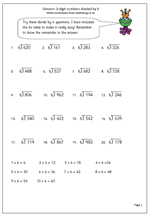# Divide 3-digit numbers by 6This is a short division page which concentrates just on dividing 3-digit numbers by 6. Short division, as its name suggests is a shortened version of the long division method where subtractions and remainders are done mentally.

The key to answering these is to have a set speech for the process.

For example: 6)457

‘How many sixes in 45?’

‘7 times 6 is 42.’

‘6 goes into 45, 7 times with 3 left over.’

Write the 7 in the answer above the 5.

Write the remainder three beside the 7 units, making 37.

‘How many sixes in 37?’

‘6 times 6 is 36.’

‘6 goes into 37, 6 times with 1 left over.’

Write the 6 in the answer in the units and write rem 1 next to it.

Division: 3-digit numbers by 6